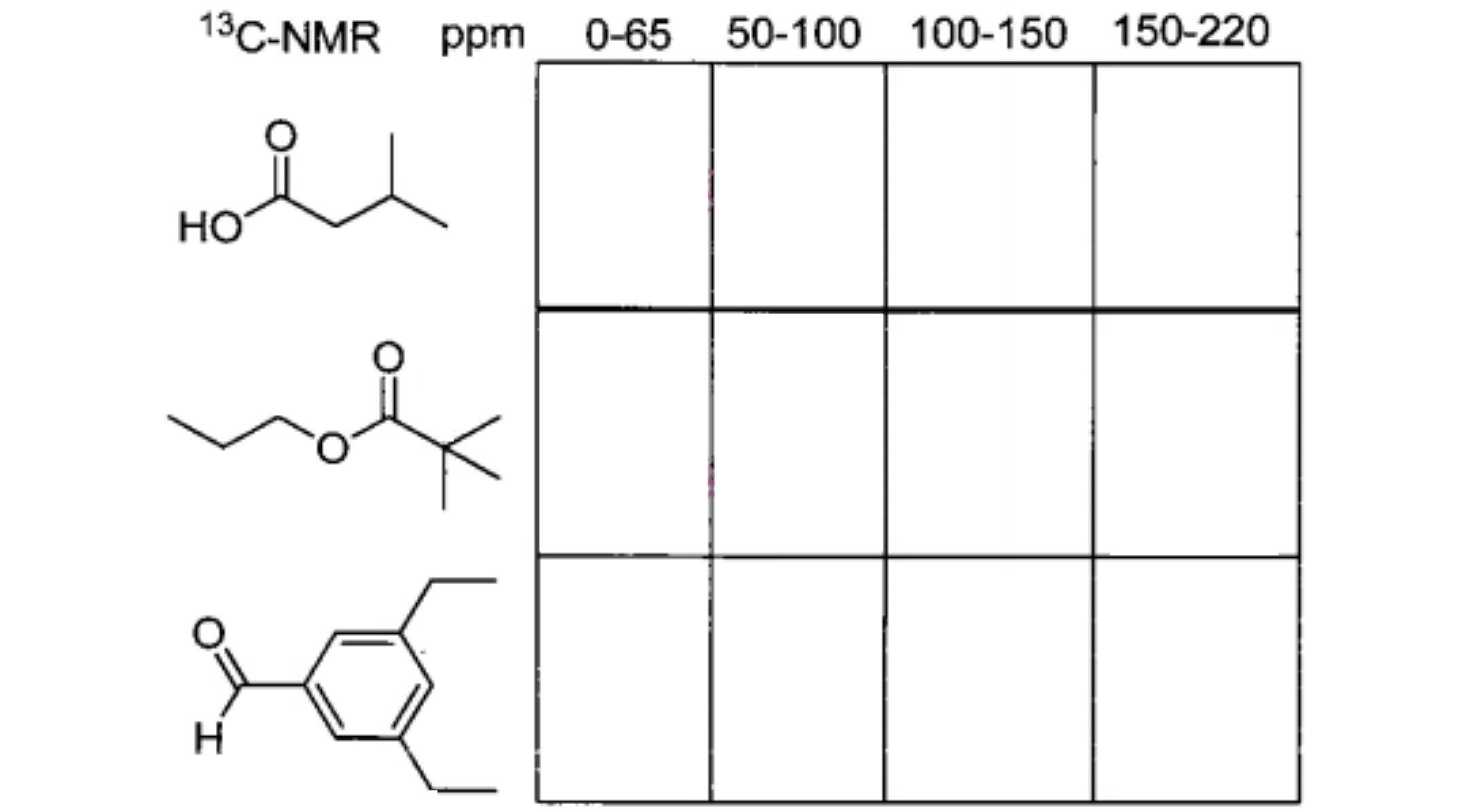Problem: How many signals would you expect to find in each range of the 13C-NMR spectrum of the following compounds? Enter a number in each box.

FREE Expert Solution
79% (160 ratings)
Problem Details

How many signals would you expect to find in each range of the 13C-NMR spectrum of the following compounds? Enter a number in each box.What scientific concept do you need to know in order to solve this problem?

Our tutors have indicated that to solve this problem you will need to apply the Carbon NMR concept. You can view video lessons to learn Carbon NMR Or if you need more Carbon NMR practice, you can also practice Carbon NMR practice problems .

What is the difficulty of this problem?

Our tutors rated the difficulty of How many signals would you expect to find in each range of t... as high difficulty.

How long does this problem take to solve?

Our expert Organic tutor, Jonathan took 11 minutes to solve this problem. You can follow their steps in the video explanation above.

What professor is this problem relevant for?

Based on our data, we think this problem is relevant for Professor Ott's class at OAKLAND.